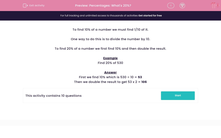# Percentages: What's 20%?

In this worksheet, students understand how to calculate 20% of a given number.This content is premium and exclusive to EdPlace subscribers.Key stage:  KS 2

Curriculum topic:   Maths and Numerical Reasoning

Curriculum subtopic:   Percentages

Difficulty level:#### Worksheet Overview

To find 10% of a number we must find 1/10 of it.

One way to do this is to divide the number by 10.

To find 20% of a number we first find 10% and then double the result.

Example

Find 20% of 530